# Wimbledon finals

Serena Williams made a successful first serve 67% 0f the time in a Wimbledon finals match against her sister Venus. If she continues to serve at the same rate the next time they play and serves 6 times in the first game, determine the probability that:

1. all six first serves will be in.
2. at least 4 first serves will be in.

p6 =  0.0905
p2 =  0.687

### Step-by-step explanation:Did you find an error or inaccuracy? Feel free to write us. Thank you!Tips to related online calculators
Looking for a statistical calculator?
Would you like to compute count of combinations?

## Related math problems and questions:

• Binomial probability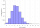What is the binominal probability that at least 4 of the 6 trials (n=6) are succeeded where φ = 0.50?
• Internet anywhere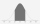In school, 60% of pupils have access to the internet at home. A group of 8 students is chosen at random. Find the probability that a) exactly 5 have access to the internet. b) At least 6 students have access to the internet
• Sick days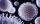In Canada, there are typically 261 working days per year. If there is a 4.9% chance that an employee takes a sick day. .. what is the probability an employee will use 17 OR MORE sick days in a year?
• Doctors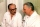The drug successfully treats 90% of cases. Calculate the probability that he will cure at least 18 patients out of 20?
• FamilyWhat is the probability that a family with 7 childrens have: exactly 6 dívek? 2 dívky and 5 boys? Consider the birth probability of a girl is 48.87% and boy 51.13%.
• The accompanyingThe accompanying table gives the probability distribution of the number of courses randomly selected student has registered Number of courses 1 2 3 4 5 6 7 Probability 0.02 0.03 0.1 0.3 0.4 - 0.01 respectively. a) Find the probability of a student registe
• RecordsRecords indicate 90% error-free. If 8 records are randomly selected, what is the probability that at least 2 records have no errors?
• Family94 boys are born per 100 girls. Determine the probability that there are two boys in a randomly selected family with three children.
• All use computerIt is reported that 72% of working women use computers at work. Choose 3 women at random, find the probability that all 3 women use a computer in their jobs.
• Probability of intersectionThree students have a probability of 0.7,0.5 and 0.4 to graduated from university respectively. What is the probability that at least one of them will be graduated?
• Probability of malaria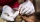A survey carried out at a certain hospital indicates that the probability that a patient tested positive for malaria is 0.6. What is the probability that two patients selected at random (i) one is negative while the other tested positive? (i) both patient
• DiceWe throw 10 times a playing dice. What is the probability that the six will fall exactly 4 times?
• Unemployment rate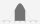Over the last 16 years, the country's unemployment rate has changed according to the following frequency table: years of unemployment: 2 5 2 3 3 1 unemploymen rate: 0.5 1 1.5 2 2.5 3 in % (percent). Determine the two-sided confidence interval for the vari
• Component fail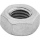There is a 90 percent chance that a particular type of component will perform adequately under high temperature conditions. If the device involved has four such components, determine the probability that the device is inoperable because exactly one of the
• Six questions testThere are six questions in the test. There are 3 answers to each - only one is correct. In order for a student to take the exam, at least four questions must be answered correctly. Alan didn't learn at all, so he circled the answers only by guessing. What
• SeedsThe germination of seeds of a certain species of carrot is 96%. What is the probability that at least 25 seeds out of 30 will germinate?
• SalesFrom statistics of sales goods, item A buy 51% of people and item B buys 59% of people. What is the probability that from 10 people buy 2 item A and 8 item B?